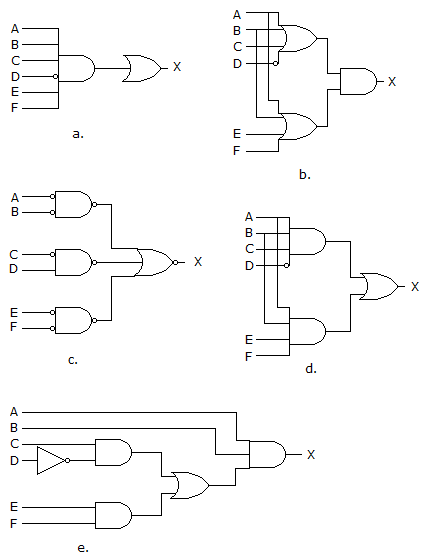# Digital Electronics - Combinational Logic Circuits - Discussion

Discussion Forum : Combinational Logic Circuits - General Questions (Q.No. 3)
3.

Which of the circuits in figure (a to d) is the sum-of-products implementation of figure (e)?a
b
c
d
Explanation:
No answer description is available. Let's discuss.
Discussion:
6 comments Page 1 of 1.

Logesh said:   7 years ago
I can't get cleared, Somebody explain it in detail.
(1)

Souradip Guha said:   7 years ago
From Fig.(e),

A.B.(C.D' + E.F)
= A.B.C.D' + A.B.E.F

Fig (a) is excluded as AND gate has 6 inputs,
Fig (b) is excluded as it is the product of the sums,
Fig (c) is excluded as OR gate has 3 AND inputs,

So,
Fig (d) is the correct answer as it is the sum of products.

Freedom said:   8 years ago
The option d is correct.

Chandrasekhar lanka said:   9 years ago
Here, at d option contain the OR gate followed by the AND gates so SOP form.

Shivanand Lecturer said:   1 decade ago
Since we get output of e ckt as (c*^d+e*f)*a.b multiply we get abc^d+abef which sum of product form.